# ISEE Upper Level Quantitative : How to multiply variables

## Example Questions

1 3 Next →

### Example Question #21 : How To Multiply Variables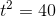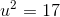Evaluate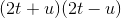.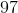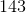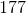Explanation:is the product of the sum and the difference of the same two binomials, so this can be rewritten, and evaluated, using the difference of squares pattern: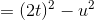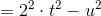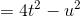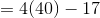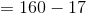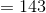1 3 Next →